Documentation

# cfplot

Visualize cash flows of financial instruments

## Syntax

``cfplot(CFlowDates,CFlowAmounts)``
``cfplot(___,Name,Value)``
``h = cfplot(___,Name,Value)``
``````[h,axes_handle] = cfplot(___,Name,Value)``````

## Description

example

````cfplot(CFlowDates,CFlowAmounts)` plots a cash flow diagram for the specified cash flow amounts (`CFlowAmounts`) and dates (`CFlowDates`). The length and orientation of each arrow correspond to the cash flow amount. ```

example

````cfplot(___,Name,Value)` plots a cash flow diagram for the specified cash flow amounts (`CFlowAmounts`), dates (`CFlowDates`), and optional name-value pair arguments.```

example

````h = cfplot(___,Name,Value)` returns the handle to the line objects used in the cash flow diagram.```

example

``````[h,axes_handle] = cfplot(___,Name,Value)``` returns the handles to the line objects and the axes using optional name-value pair arguments. ```

## Examples

collapse all

Define `CFlowAmounts` and `CFlowDates` using the `cfamounts` function.

```CouponRate = [0.06; 0.05; 0.03]; Settle = '03-Jun-1999'; Maturity = ['15-Aug-2000';'15-Dec-2000';'15-Jun-2000']; Period = [1; 2; 2]; Basis = [1; 0; 0]; [CFlowAmounts, CFlowDates] = cfamounts(... CouponRate, Settle, Maturity, Period, Basis)```
```CFlowAmounts = 3×5 -4.8000 6.0000 106.0000 NaN NaN -2.3352 2.5000 2.5000 2.5000 102.5000 -1.4011 1.5000 1.5000 101.5000 NaN ```
```CFlowDates = 3×5 730274 730347 730713 NaN NaN 730274 730286 730469 730652 730835 730274 730286 730469 730652 NaN ```

Plot all cash flows on the same axes, and label the first two.

`cfplot(CFlowDates, CFlowAmounts, 'ShowAmnt', [1 2])`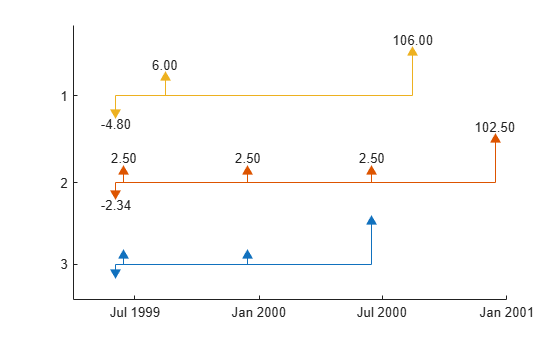Group the second and third cash flows.

```figure; cfplot(CFlowDates, CFlowAmounts, 'Groups', {[2 3]}, 'ShowAmnt', 1);```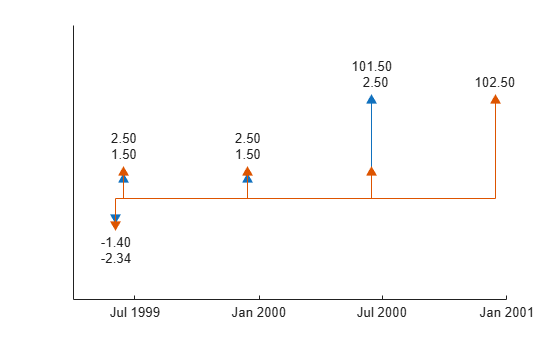Format the date axis and place ticks on actual cash flow dates.

```figure; cfplot(CFlowDates, CFlowAmounts, 'Groups', {[2 3]}, 'ShowAmnt', 1, ... 'DateFormat', 6, 'DateSpacing', 100);```Stack the cash flow arrows occurring on the same dates.

```figure; cfplot(CFlowDates, CFlowAmounts, 'Groups', {[2 3]}, 'ShowAmnt', 1, ... 'DateFormat', 6, 'DateSpacing', 100, 'Stacked', 1);```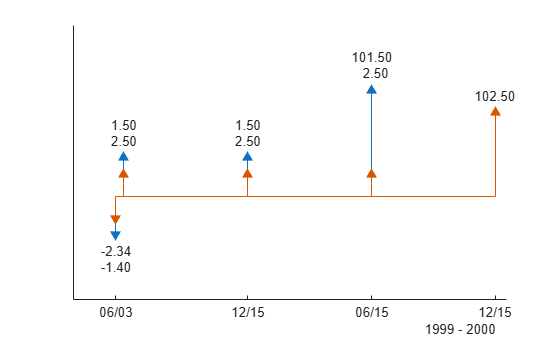Form subplots of multiple groups and add titles using axes handles.

```figure; [h, axes_handle] = cfplot(CFlowDates, CFlowAmounts, ... 'Groups', { [2 3]}, 'ShowAmnt', 1, 'Stacked', 2, ... 'DateSpacing', [1 60 2 100], 'DateFormat', [1 12 2 6]); title(axes_handle(1), 'Group 1', 'FontWeight', 'bold'); title(axes_handle(2), 'Group 2', 'FontWeight', 'bold');```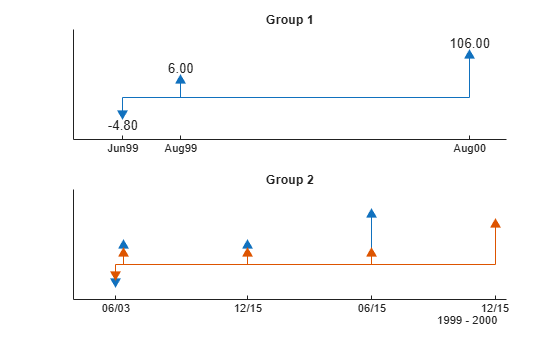Define `CFlowDates` using `datetime` input and plot the cash flow.

```CouponRate = [0.06; 0.05; 0.03]; Settle = '03-Jun-1999'; Maturity = ['15-Aug-2000';'15-Dec-2000';'15-Jun-2000']; Period = [1; 2; 2]; Basis = [1; 0; 0]; [CFlowAmounts, CFlowDates] = cfamounts(... CouponRate, Settle, Maturity, Period, Basis); cfplot(datetime(CFlowDates,'ConvertFrom','datenum','Locale','en_US'), CFlowAmounts, 'ShowAmnt', [1 2])```Define the swap using the `swapbyzero` function.

```Settle = datenum('08-Jun-2010'); RateSpec = intenvset('Rates', [.005 .0075 .01 .014 .02 .025 .03]',... 'StartDates',Settle, 'EndDates',{'08-Dec-2010','08-Jun-2011',... '08-Jun-2012','08-Jun-2013','08-Jun-2015','08-Jun-2017',... '08-Jun-2020'}'); Maturity = datenum('15-Sep-2020'); LegRate = [.025 50]; LegType = [1 0]; % fixed/floating LatestFloatingRate = .005; [Price, SwapRate, AI, RecCF, RecCFDates, PayCF,PayCFDates] = ... swapbyzero(RateSpec, LegRate, Settle, Maturity,'LegType',LegType,... 'LatestFloatingRate',LatestFloatingRate)```
```Price = -6.7258 ```
```SwapRate = NaN ```
```AI = 1.4575 ```
```RecCF = 1×12 -1.8219 2.5000 2.5000 2.5000 2.5000 2.5000 2.5000 2.5000 2.5000 2.5000 2.5000 102.5000 ```
```RecCFDates = 1×12 734297 734396 734761 735127 735492 735857 736222 736588 736953 737318 737683 738049 ```
```PayCF = 1×12 -0.3644 0.5000 1.4048 1.9823 2.8436 3.2842 3.8218 4.1733 4.5164 4.4666 4.8068 104.6743 ```
```PayCFDates = 1×12 734297 734396 734761 735127 735492 735857 736222 736588 736953 737318 737683 738049 ```

Define `CFlowDates` and `CFlowAmounts` for the swap and generate a cash flow plot using `cfplot`.

```CFlowDates = [PayCFDates;RecCFDates]; CFlowAmounts = [-PayCF;RecCF]; cfplot(CFlowDates, CFlowAmounts, 'Groups', {[1 2]}); xlabel('Numeric Cash Flow Dates');```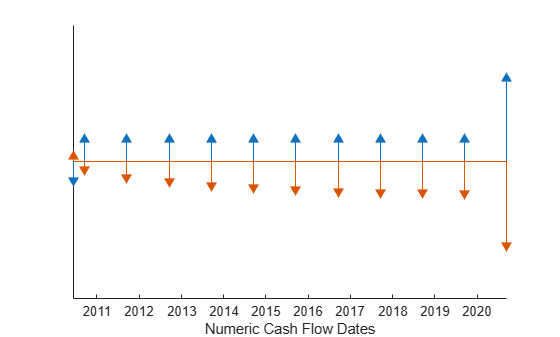## Input Arguments

collapse all

Matrix of serial date numbers or datetime arrays for cash flows, specified as a `NINST`-by-`(Number of cash flows)` matrix of cash flow dates in date numbers, with empty entries padded with `NaN`s.

Each row of the `CFlowDates` matrix represents an instrument so that `CFlowDates(k,:)` is the vector of cash flow dates for the `k`th instrument. Rows are padded with trailing `NaN`s if the number of cash flows is not the same for all instruments.

`cfamounts` can be used to generate `CFlowDates`.

Data Types: `double`

Matrix of cash flow amounts, specified as a `NINST`-by-`(Number of cash flows)` matrix of cash flow amounts, with empty entries padded with `NaN`s. The `CFlowAmounts` matrix must be the same size as `CFlowDates`.

`cfamounts` can be used to generate `CFlowAmounts`.

Data Types: `double`

### Name-Value Pair Arguments

Specify optional comma-separated pairs of `Name,Value` arguments. `Name` is the argument name and `Value` is the corresponding value. `Name` must appear inside quotes. You can specify several name and value pair arguments in any order as `Name1,Value1,...,NameN,ValueN`.

Example: ```cfplot(CFlowDates,CFlowAmounts,'Groups',{[2 3]},'ShowAmnt',1,'DateFormat',6,'DateSpacing',100)```

Group cash flows, specified as the comma-separated pair consisting of `'Groups'` and the following values:

• `'off'` — Show all instruments in one set of axes, arranged from top to bottom.

• `'individual'` — Generate subplots and plot each instrument in its own axis.

• `GRP` — Cell array of instrument groups, `{Group1, Group2,... }`. This generates subplots and plots each group in each axis. When specifying `{Group1, Group2,... }`, each Group must be mutually exclusive vectors of `INSTIndex`. Unspecified instruments are not shown in the grouped plot.

Data Types: `char` | `cell`

Stack arrows if the cash flows are in the same direction on the same day, specified as the comma-separated pair consisting of `'Stacked'` and the following values:

• `'off'` — For all groups, all arrows originate from the horizontal line.

• `'all'` — For all groups, arrows are stacked if the cash flows are in the same direction on the same day.

• `'GRPIndex'` — For specified groups, arrows are stacked if the cash flows are in the same direction on the same day.

Data Types: `char`

Show amount on the arrows, specified as the comma-separated pair consisting of `'ShowAmnt'` and the following values:

• `'off'` — Hide cash flow amounts on arrows.

• `'all'` — Show cash flow amounts on arrows.

• [`INSTIndex` or `GRPIndex`] — Show cash flow amounts for the specified vector of instruments (when `'Groups'` is `'off'`) or groups.

Data Types: `char` | `cell`

Control for data spacing, specified as the comma-separated pair consisting of `'DateSpacing'` and the following values:

• `'off'` — The date axis ticks are spaced regularly.

• `TickDateSpace` — The date axis ticks are placed on actual cash flow dates. The ticks skip some cash flows if they are less than `TickDateSpace` apart.

Data Types: `char` | `double`

Date format, specified as the comma-separated pair consisting of `'DateFormat'` and the following values:

• `'off'` — The date axis tick labels are in date numbers.

• `DateFormNum` — The date format number (`2` = `'mm/dd/yy'`, 6 = `'mm/dd'`, and `10` = `'yyyy'`). Additional values for `DateFormNum` are as follows:

`DateFormNum`Example
`2` 03/01/00
`3`Mar
`5`03
`6`03/01
`7`01
`8`Wed
`9`W
`10`2000
`11`00
`12`Mar00
`17`Q1–00
`18`Q1
`19`01/03
`20`01/03/00
`27`Q1–2000
`28`Mar2000
`29`2000–03–01

Data Types: `char` | `double`

## Output Arguments

collapse all

Handles to line objects, returned as a `NINST`-by-`3` matrix of handles to line objects, containing `[``hLines`, `hUArrowHead`, `hDArrowHead``]` where:

• `hLines` — Horizontal and vertical lines used in the cash flow diagram

• `hUArrowHead` — "Up" arrowheads

• `hDArrowHead` — "Down" arrowheads

Handles to axes for the plot or subplots, returned as a (```Number of axes```)-by-`1` vector of handles to axes.